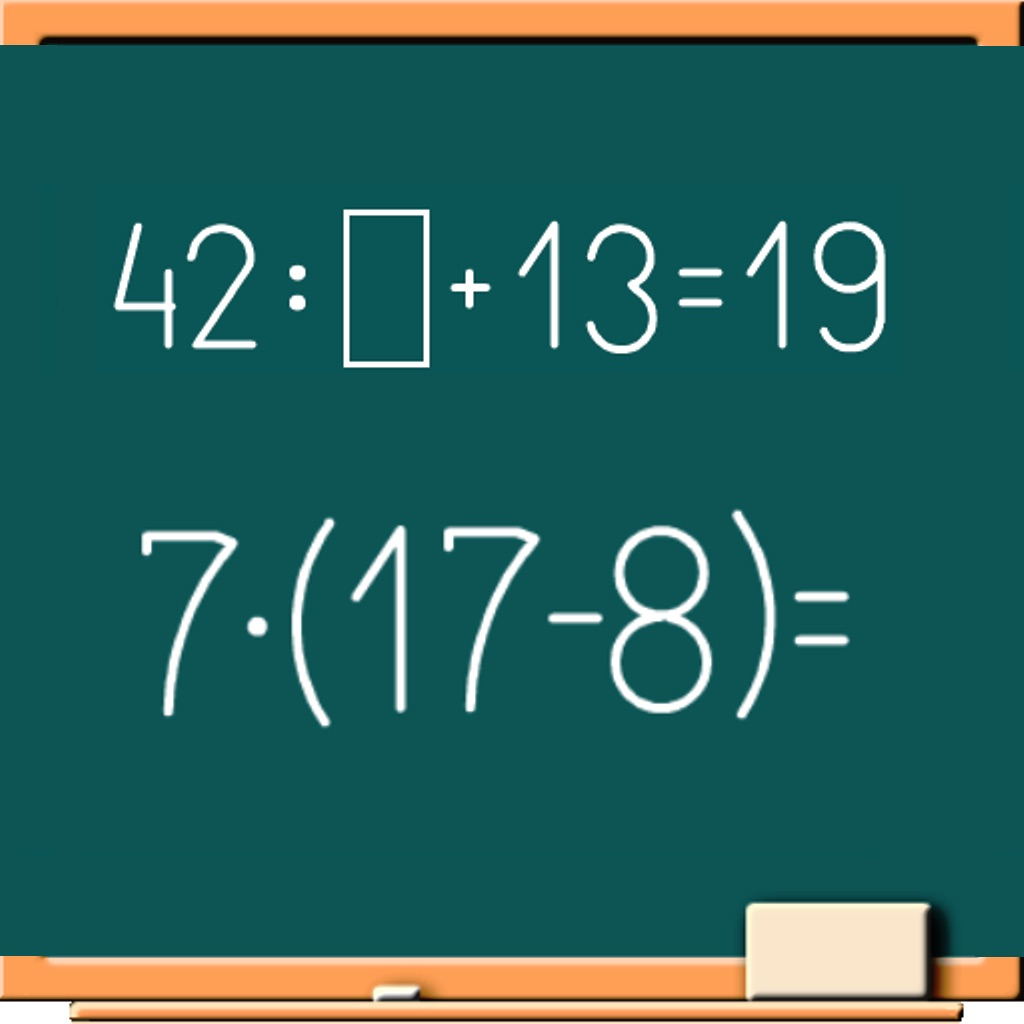Math on Chalkboard# Math on Chalkboard

## Hovhannes Nshanyan

\$3.99
0

0 Ratings

Release Date

2017-01-10

Size

19.1 MB

### Screenshots for iPhone

iPhone
Math on Chalkboard Description
Math application "Math on chalkboard" is presented as an animated game that helps you to enhance mental arithmetic skills, facilitates the memorization of multiplication and division table. Good animation gives you a feeling of being in the classroom, in front of the board.
The application consists of 5 age categories with relevant topics:

Preschool (numbers up to 10)
• Comparison
• Number sequences

1st grade (numbers up to 20)
• Comparison
• Number sequences

• Comparison
• Multiplication and division table
• Mixed expressions
• Number sequences

• Comparison
• Mixed expressions
• Number sequences

Children 12+ and Adults(negative and positive numbers)
• Mixed expressions with two-digit numbers
• Comparison two-digit numbers
• Mixed expressions with three-digit numbers
• Comparison three-digit numbers
• Number sequences

"Addition and subtraction" are mathematical calculations with bit transition or without transition.
"Comparison" includes a comparison of the numbers, as well as results of mathematical calculations.
"Multiplication and division table" will allow children to learn the multiplication table, adults may also refresh their knowledge of math.
"Mixed expressions" provides mathematical exercises with use of plus, minus, multiplication and division, as well as expressions with brackets and examples in few actions.
"Number sequences" is a number series where you must find the pattern and insert the missing number.

All the math exercises are grouped by levels. The higher is level, the more difficult is calculation. To move to the next level you must correctly solve all the exercises of the current level.
After selecting the age category and topic, you can move on to practical exercises ("Practice") or test tasks ("Tests").

The section "Practice" contains many different kinds of mathematical exercises that help to develop the skills of oral accounts. It is job training which helps you to improve the knowledge of math, and then easily pass the tests of this application.
In the section "Tests" for each exercise 15 seconds are given. If the result is not entered within 15 seconds, the answer is considered incorrect. This highly improves the ability of quickly finding solutions of mathematical expressions.

The scoreboard indicates the total number of exercises on the current level, and the number of correct and incorrect answers.
The application is made in the form of the simulator. Regular, repeated exercise will give good result in mastering arithmetic calculations such as addition, subtraction, multiplication, division, and will help quickly learn the multiplication and division table. Because for many arithmetic and mathematical tasks requires excellent knowledge of multiplication and division tables.

Features of the application:
• 5 age categories;
• more than 3,000 math exercises on different topics;
• multi-level system with increasing difficulty;
• animated chalkboard for self-training;
• sound effects;
• multi-language support;
• statistics showing the number of levels on the topics.

Supported languages:
English, Armenian, Spanish, Italian, Chinese, German, Portuguese, Russian, Ukrainian, French, Korean, Japanese, Arabic.
Math on Chalkboard 1.0 Update
2017-01-10 Version History
More
Price:
\$3.99
Version:
1.0
Size:
19.1 MB
Release Date:
2017-01-10
Developer:
Hovhannes Nshanyan
Language:
Chinese English French German Italian Japanese Korean Portuguese Russian Spanish
More
You May Also Like
Developer Apps